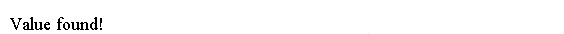# Java Tutorial - Java For

Java `for` loop statement provides a powerful way of writing loop statement.

The simplest form of the `for` loop is shown here:

```   for(initialization; condition; iteration)
statement;
```

Java for loop statement has three parts:

• `initialization` sets a loop control variable to an initial value.
• `condition` is a `Boolean` expression that tests the loop control variable. If `condition` is `true`, the for loop continues to iterate. If `condition` is `false`, the loop terminates.
• The `iteration` determines how the loop control variable is changed each time the loop iterates.

Here is a short program that illustrates the for loop. `i` is the loop control variable and `i` is initialized to zero in the initialization. At the start of each iteration, the conditional test `x < 10` is performed. If the outcome of this test is true, the `println()` statement is executed, and then the iteration portion of the loop is executed. This process continues until the conditional test is `false`.

```public class Main {
public static void main(String args[]) {
int i;

for (i = 0; i < 10; i = i + 1)
System.out.println("This is i: " + i);
}
}
```

This program generates the following output: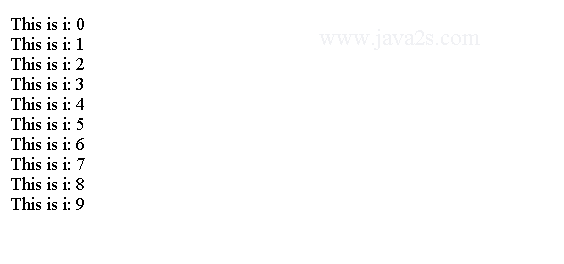## Example

The following code writes the code logic from above again but loops reversively:

```
public class Main {
public static void main(String args[]) {
for (int n = 10; n > 0; n--)
System.out.println("n:" + n);
}
}  ]]>
```

The output: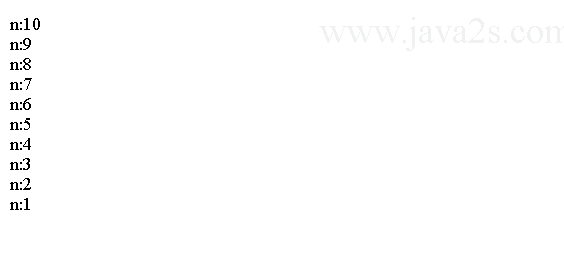## Example 2

Here is a program that tests for prime numbers using `for` loop statement.

```
public class Main {
public static void main(String args[]) {
int num;/* w  w  w.java2 s  . com*/
boolean isPrime = true;
num = 50;
for (int i = 2; i <= num / 2; i++) {
if ((num % i) == 0) {
isPrime = false;
break;
}
}
if (isPrime)
System.out.println("Prime");
else
System.out.println("Not Prime");

}
}
```

The output: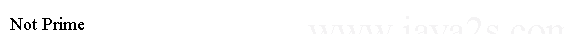## Example 3

Java allows two or more variables to control a `for` loop. And you can include multiple statements in both the initialization and iteration portions of the `for` loop. Each statement is separated from the next by a comma. Here is an example:

```
public class Main {
public static void main(String args[]) {
//w w w . j  a  v  a2 s  . co m
for (int a = 1, b = 4; a < b; a++, b--) {
System.out.println("a = " + a);
System.out.println("b = " + b);

}
}
}
```

The program generates the following output: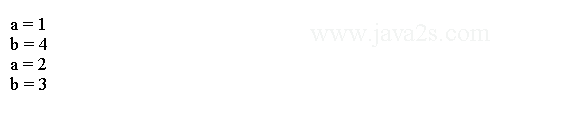## Example 4

The three sections of the `for` can be used for any purpose and parts of the `for` loop can be empty.

```
public class Main {
public static void main(String args[]) {
int i = 0;/*from  w w w. j  a va2  s  .c o m*/
boolean done = false;
for (; !done;) {
System.out.println("i is " + i);
if (i == 10)
done = true;
i++;

}
}
}
```

The output: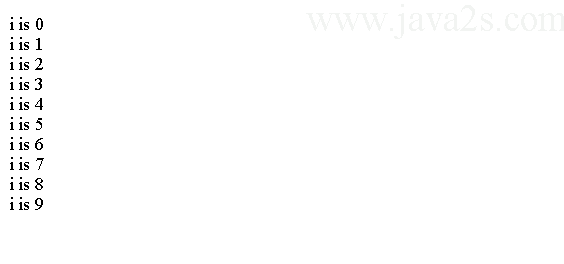## Example 5

`for` loop can be nested to produce powerful logic, for example, we can use nested `for` loop to iterate a two-dimensional array. For example, here is a program that nests `for` loops:

```
public class Main {
public static void main(String args[]) {
for (int i = 0; i < 10; i++) {
for (int j = i; j < 10; j++)
System.out.print(".");
System.out.println();
}
}
}
```

The output produced by this program is shown here: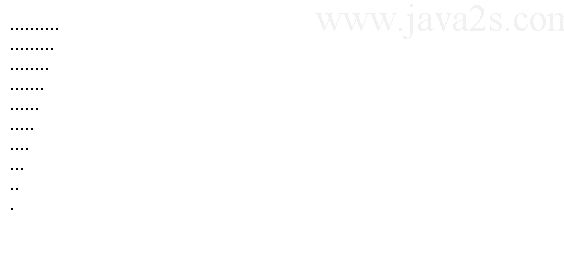## Java for each loop

The `for each` loop iterates elements in a sequence without using the loop counter.

The syntax of `for each` loop is:

```for (type variable_name:array){

}
```

The `type` must be compatible with the `array` type.

The following code shows how to use for each loop.

```public class Main {
public static void main(String args[]) {
String[] arr = new String[]{"java2s.com","a","b","c"};
for(String s:arr){
System.out.println(s);
}
}
}
```

The output:## Example 6

The following code uses the `for-each` style loop to iterate a two-dimensional array.

```public class Main {
public static void main(String args[]) {
int sum = 0;/* w  w w.  ja  va2  s  . c o  m*/
int nums[][] = new int;
for (int i = 0; i < 3; i++){
for (int j = 0; j < 5; j++){
nums[i][j] = (i + 1) * (j + 1);
}
}
// use for-each for to display and sum the values
for (int x[] : nums) {
for (int y : x) {
System.out.println("Value is: " + y);
sum += y;
}
}
System.out.println("Summation: " + sum);
}
}
```

The output from this program is shown here: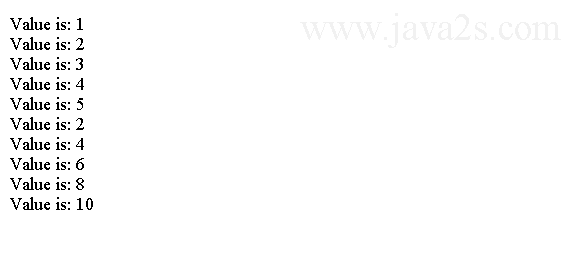## Example 7

`for-each` style loop is useful when searching an element in an array.

```public class Main {
public static void main(String args[]) {
int nums[] = { 6, 8, 3, 7, 5, 6, 1, 4 };
int val = 5;//from   ww w.j a  v a 2s . c  om
boolean found = false;
// use for-each style for to search nums for val
for (int x : nums) {
if (x == val) {
found = true;
break;
}
}
if (found)
System.out.println("Value found!");
}
}
```

The code above generates the following result.